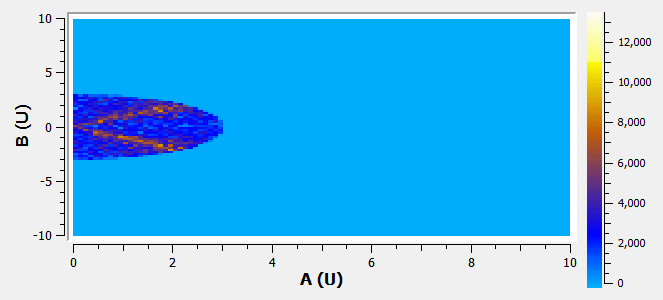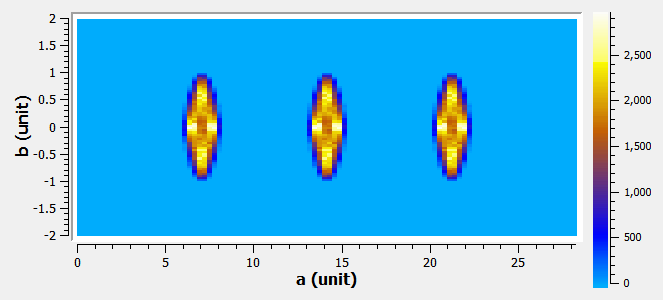$$\renewcommand\AA{\unicode{x212B}}$$

# BinMD v1¶

## Summary¶

Take a MDEventWorkspace and bin into into a dense, multi-dimensional histogram workspace (MDHistoWorkspace).

## Properties¶

Name

Direction

Type

Default

Description

InputWorkspace

Input

IMDWorkspace

Mandatory

An input MDWorkspace.

AxisAligned

Input

boolean

True

Perform binning aligned with the axes of the input MDEventWorkspace?

AlignedDim0

Input

string

Binning parameters for the 0th dimension. Enter it as a comma-separated list of values with the format: ‘name,minimum,maximum,number_of_bins’. Leave blank for NONE.

AlignedDim1

Input

string

Binning parameters for the 1th dimension. Enter it as a comma-separated list of values with the format: ‘name,minimum,maximum,number_of_bins’. Leave blank for NONE.

AlignedDim2

Input

string

Binning parameters for the 2th dimension. Enter it as a comma-separated list of values with the format: ‘name,minimum,maximum,number_of_bins’. Leave blank for NONE.

AlignedDim3

Input

string

Binning parameters for the 3th dimension. Enter it as a comma-separated list of values with the format: ‘name,minimum,maximum,number_of_bins’. Leave blank for NONE.

AlignedDim4

Input

string

Binning parameters for the 4th dimension. Enter it as a comma-separated list of values with the format: ‘name,minimum,maximum,number_of_bins’. Leave blank for NONE.

AlignedDim5

Input

string

Binning parameters for the 5th dimension. Enter it as a comma-separated list of values with the format: ‘name,minimum,maximum,number_of_bins’. Leave blank for NONE.

BasisVector0

Input

string

Description of the basis vector of the 0th output dimension.Format: ‘name, units, x,y,z,..’. name : string for the name of the output dimension. units : string for the units of the output dimension. x,y,z,…: vector definining the basis in the input dimensions space. Leave blank for NONE.

BasisVector1

Input

string

Description of the basis vector of the 1th output dimension.Format: ‘name, units, x,y,z,..’. name : string for the name of the output dimension. units : string for the units of the output dimension. x,y,z,…: vector definining the basis in the input dimensions space. Leave blank for NONE.

BasisVector2

Input

string

Description of the basis vector of the 2th output dimension.Format: ‘name, units, x,y,z,..’. name : string for the name of the output dimension. units : string for the units of the output dimension. x,y,z,…: vector definining the basis in the input dimensions space. Leave blank for NONE.

BasisVector3

Input

string

Description of the basis vector of the 3th output dimension.Format: ‘name, units, x,y,z,..’. name : string for the name of the output dimension. units : string for the units of the output dimension. x,y,z,…: vector definining the basis in the input dimensions space. Leave blank for NONE.

BasisVector4

Input

string

Description of the basis vector of the 4th output dimension.Format: ‘name, units, x,y,z,..’. name : string for the name of the output dimension. units : string for the units of the output dimension. x,y,z,…: vector definining the basis in the input dimensions space. Leave blank for NONE.

BasisVector5

Input

string

Description of the basis vector of the 5th output dimension.Format: ‘name, units, x,y,z,..’. name : string for the name of the output dimension. units : string for the units of the output dimension. x,y,z,…: vector definining the basis in the input dimensions space. Leave blank for NONE.

Translation

Input

dbl list

Coordinates in the INPUT workspace that corresponds to (0,0,0) in the OUTPUT workspace. Enter as a comma-separated string. Default: 0 in all dimensions (no translation).

OutputExtents

Input

dbl list

The minimum, maximum edges of space of each dimension of the OUTPUT workspace, as a comma-separated list

OutputBins

Input

int list

The number of bins for each dimension of the OUTPUT workspace.

NormalizeBasisVectors

Input

boolean

True

Normalize the given basis vectors to unity. If true, then a distance of 1 in the INPUT dimensions = 1 in the OUTPUT dimensions. If false, then a distance of norm(basis_vector) in the INPUT dimension = 1 in the OUTPUT dimensions.

ForceOrthogonal

Input

boolean

False

Force the input basis vectors to form an orthogonal coordinate system. Only works in 3 dimension!

ImplicitFunctionXML

Input

string

XML string describing the implicit function determining which bins to use.

IterateEvents

Input

boolean

True

Alternative binning method where you iterate through every event, placing them in the proper bin. This may be faster for workspaces with few events and lots of output bins.

Parallel

Input

boolean

False

Temporary parameter: true to run in parallel. This is ignored for file-backed workspaces, where running in parallel makes things slower due to disk thrashing.

TemporaryDataWorkspace

Input

IMDHistoWorkspace

An input MDHistoWorkspace used to accumulate results from multiple MDEventWorkspaces. If unspecified a blank MDHistoWorkspace will be created.

OutputWorkspace

Output

Workspace

Mandatory

A name for the output MDHistoWorkspace.

## Description¶

This algorithm performs dense binning of the events in multiple dimensions of an input MDWorkspace and places them into a dense MDHistoWorkspace with 1-4 dimensions.

The input MDWorkspace may have more dimensions than the number of output dimensions. The names of the dimensions in the DimX, etc. parameters are used to find the corresponding dimensions that will be created in the output.

An ImplicitFunction can be defined using the ImplicitFunctionXML parameter; any points NOT belonging inside of the ImplicitFunction will be set as NaN (not-a-number).

### Axis-Aligned Binning¶

This is binning where the output axes are aligned with the original workspace. Specify each of the AlignedDim0, etc. parameters with these values, separated by commas:

• First, the name of the dimension in the original workspace

• Next, the start and end position along that dimension

• Finally, the number of bins to use in that dimensions.

If you specify fewer output dimensions, then events in the remaining dimensions will be integrated along all space. If you wish to integrate only within a range, then specify the start and end points, with only 1 bin.

### Non-Axis Aligned Binning¶

This allows rebinning to a new arbitrary space, with rotations, translations, or skewing. This is given by a set of basis vectors and other parameters

The BasisVector0… parameters allow you to specify the basis vectors relating the input coordinates to the output coordinates. They are string with these parameters, separated by commas: ‘name, units, x,y,z,..,’:

• Name: of the new dimension

• Units: string giving the units

• x, y, z, etc.: a vector, matching the number of dimensions, giving the direction of the basis vector

The Translation parameter defines the translation between the spaces. The coordinates are given in the input space dimensions, and they correspond to 0,0,0 in the output space.

The OutputExtents parameter specifies the min/max coordinates in the output coordinate space. For example, if you the output X to range from -5 to 5, and output Y to go from 0 to 10, you would have: “-5,5, 0,10”.

The OutputBins parameter specifies how many bins to use in the output workspace for each dimension. For example, “10,20,30” will make 10 bins in X, 20 bins in Y and 30 bins in Z.

If the NormalizeBasisVectors parameter is True, then the distances in the input space are the same as in the output space (no scaling).

• For example, if BasisVector0=(1,1), a point at (1,1) transforms to $$x=\sqrt{2}$$, which is the same as the distance in the input dimension.

If the NormalizeBasisVectors parameter is False, then the algorithm will take into account the length of each basis vector.

• For example, if BasisVector0=(1,1), a point at (1,1) transforms to $$x=1$$. The distance was scaled by $$1/\sqrt{2}$$

Finally, the ForceOrthogonal parameter will modify your basis vectors if needed to make them orthogonal to each other. Only works in 3 dimensions!

### Binning a MDHistoWorkspace¶

It is possible to rebin a MDHistoWorkspace. Each MDHistoWorkspace holds a reference to the MDWorkspace that created it, as well as the coordinate transformation that was used. In this case, the rebinning is actually performed on the original MDWorkspace, after suitably transforming the basis vectors.

Only the non-axis aligned binning method can be performed on a MDHistoWorkspace! Of course, your basis vectors can be aligned with the dimensions, which is equivalent.

For more details on the coordinate transformations applied in this case, please see BinMD Coordinate Transformations.

## Usage¶

Axis Aligned Example

mdws = CreateMDWorkspace(Dimensions=3, Extents='-10,10,-10,10,-10,10', Names='A,B,C', Units='U,U,U')
FakeMDEventData(InputWorkspace=mdws, PeakParams='500000,0,0,0,3')
binned_ws = BinMD(InputWorkspace=mdws, AlignedDim0='A,0,10,100', AlignedDim1='B,-10,10,100', AlignedDim2='C,-10,10,100')
print("Number of events = {}".format(binned_ws.getNEvents()))


Output:

Number of events = 250334


The output looks like the following in the Sliceviewer:Non Axis Aligned Example

import math
mdws = CreateMDWorkspace(Dimensions=3, Extents='-10,10,-10,10,-10,10', Names='A,B,C', Units='U,U,U')
FakeMDEventData(InputWorkspace=mdws, PeakParams='100000,-5,-5,0,1')
FakeMDEventData(InputWorkspace=mdws, PeakParams='100000,0,0,0,1')
FakeMDEventData(InputWorkspace=mdws, PeakParams='100000,5,5,0,1')
binned_ws = BinMD(InputWorkspace=mdws, AxisAligned=False, BasisVector0='a,unit,1,1,0',BasisVector1='b,unit,-1,1,0',BasisVector2='c,unit,0,0,1',NormalizeBasisVectors=True,Translation=[-10,-10,0], OutputExtents=[0,math.sqrt(2*20*20),-2,2,-10,10], OutputBins=[100, 100, 1] )
print("Number of events = {}".format(binned_ws.getNEvents()))


Output:

Number of events = 300000


The output looks like the following in the Sliceviewer:Accumulation Example

binned_ws=None
for x in range(3):
mdws = CreateMDWorkspace(Dimensions=2, Extents='-10,10,-10,10', Names='A,B', Units='U,U')
FakeMDEventData(InputWorkspace=mdws, PeakParams='500000,'+str(x)+',0,3')
binned_ws = BinMD(InputWorkspace=mdws, AlignedDim0='A,-10,10,100', AlignedDim1='B,-10,10,100', TemporaryDataWorkspace=binned_ws)
print("Number of events = {}".format(binned_ws.getNEvents()))


Output:

Number of events = 1500000


Categories: AlgorithmIndex | MDAlgorithms\Slicing